This book is archived and will be removed July 6, 2022. Please use the updated version.

Inductance

# 97 Oscillations in an LC Circuit

### Learning Objectives

By the end of this section, you will be able to:

• Explain why charge or current oscillates between a capacitor and inductor, respectively, when wired in series
• Describe the relationship between the charge and current oscillating between a capacitor and inductor wired in series

It is worth noting that both capacitors and inductors store energy, in their electric and magnetic fields, respectively. A circuit containing both an inductor (L) and a capacitor (C) can oscillate without a source of emf by shifting the energy stored in the circuit between the electric and magnetic fields. Thus, the concepts we develop in this section are directly applicable to the exchange of energy between the electric and magnetic fields in electromagnetic waves, or light. We start with an idealized circuit of zero resistance that contains an inductor and a capacitor, an LC circuit.

An LC circuit is shown in (Figure). If the capacitor contains a chargebefore the switch is closed, then all the energy of the circuit is initially stored in the electric field of the capacitor ((Figure)(a)). This energy is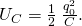When the switch is closed, the capacitor begins to discharge, producing a current in the circuit. The current, in turn, creates a magnetic field in the inductor. The net effect of this process is a transfer of energy from the capacitor, with its diminishing electric field, to the inductor, with its increasing magnetic field.

(a–d) The oscillation of charge storage with changing directions of current in an LC circuit. (e) The graphs show the distribution of charge and current between the capacitor and inductor.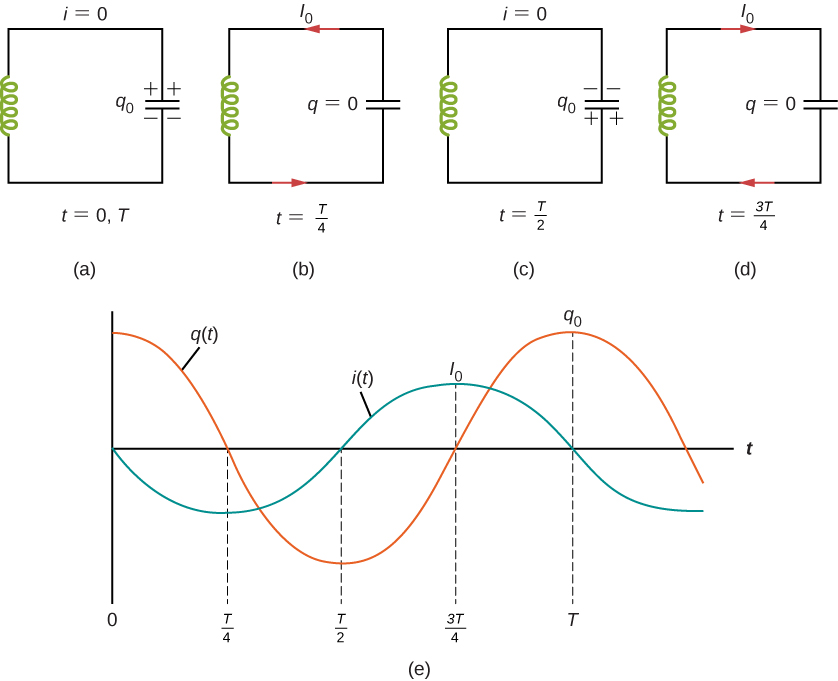In (Figure)(b), the capacitor is completely discharged and all the energy is stored in the magnetic field of the inductor. At this instant, the current is at its maximum value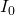and the energy in the inductor is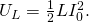Since there is no resistance in the circuit, no energy is lost through Joule heating; thus, the maximum energy stored in the capacitor is equal to the maximum energy stored at a later time in the inductor: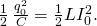At an arbitrary time when the capacitor charge is q(t) and the current is i(t), the total energy U in the circuit is given by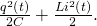Because there is no energy dissipation,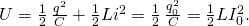After reaching its maximum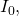the current i(t) continues to transport charge between the capacitor plates, thereby recharging the capacitor. Since the inductor resists a change in current, current continues to flow, even though the capacitor is discharged. This continued current causes the capacitor to charge with opposite polarity. The electric field of the capacitor increases while the magnetic field of the inductor diminishes, and the overall effect is a transfer of energy from the inductor back to the capacitor. From the law of energy conservation, the maximum charge that the capacitor re-acquires is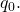However, as (Figure)(c) shows, the capacitor plates are charged opposite to what they were initially.

When fully charged, the capacitor once again transfers its energy to the inductor until it is again completely discharged, as shown in (Figure)(d). Then, in the last part of this cyclic process, energy flows back to the capacitor, and the initial state of the circuit is restored.

We have followed the circuit through one complete cycle. Its electromagnetic oscillations are analogous to the mechanical oscillations of a mass at the end of a spring. In this latter case, energy is transferred back and forth between the mass, which has kinetic energy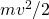, and the spring, which has potential energy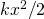. With the absence of friction in the mass-spring system, the oscillations would continue indefinitely. Similarly, the oscillations of an LC circuit with no resistance would continue forever if undisturbed; however, this ideal zero-resistance LC circuit is not practical, and any LC circuit will have at least a small resistance, which will radiate and lose energy over time.

The frequency of the oscillations in a resistance-free LC circuit may be found by analogy with the mass-spring system. For the circuit,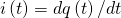, the total electromagnetic energy U is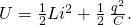For the mass-spring system,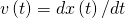, the total mechanical energy E is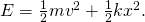The equivalence of the two systems is clear. To go from the mechanical to the electromagnetic system, we simply replace m by L, v by i, k by 1/C, and x by q. Now x(t) is given by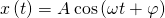where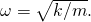Hence, the charge on the capacitor in an LC circuit is given by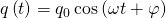where the angular frequency of the oscillations in the circuit is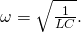Finally, the current in the LC circuit is found by taking the time derivative of q(t):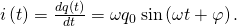The time variations of q and I are shown in (Figure)(e) for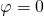.

An LC Circuit In an LC circuit, the self-inductance is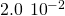H and the capacitance is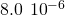F. At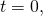all of the energy is stored in the capacitor, which has charge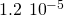C. (a) What is the angular frequency of the oscillations in the circuit? (b) What is the maximum current flowing through circuit? (c) How long does it take the capacitor to become completely discharged? (d) Find an equation that represents q(t).

Strategy The angular frequency of the LC circuit is given by (Figure). To find the maximum current, the maximum energy in the capacitor is set equal to the maximum energy in the inductor. The time for the capacitor to become discharged if it is initially charged is a quarter of the period of the cycle, so if we calculate the period of the oscillation, we can find out what a quarter of that is to find this time. Lastly, knowing the initial charge and angular frequency, we can set up a cosine equation to find q(t).

Solution

1. From (Figure), the angular frequency of the oscillations is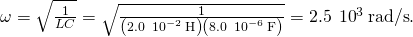2. The current is at its maximumwhen all the energy is stored in the inductor. From the law of energy conservation,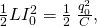so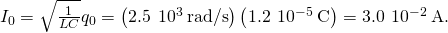This result can also be found by an analogy to simple harmonic motion, where current and charge are the velocity and position of an oscillator.

3. The capacitor becomes completely discharged in one-fourth of a cycle, or during a time T/4, where T is the period of the oscillations. Since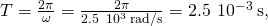the time taken for the capacitor to become fully discharged is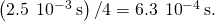4. The capacitor is completely charged atso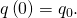Using (Figure), we obtain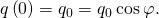Thus,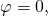and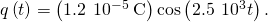Significance The energy relationship set up in part (b) is not the only way we can equate energies. At most times, some energy is stored in the capacitor and some energy is stored in the inductor. We can put both terms on each side of the equation. By examining the circuit only when there is no charge on the capacitor or no current in the inductor, we simplify the energy equation.

Check Your Understanding The angular frequency of the oscillations in an LC circuit is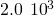rad/s. (a) If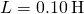, what is C? (b) Suppose that atall the energy is stored in the inductor. What is the value of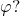(c) A second identical capacitor is connected in parallel with the original capacitor. What is the angular frequency of this circuit?

a.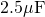; b.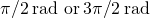; c.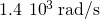### Summary

• The energy transferred in an oscillatory manner between the capacitor and inductor in an LC circuit occurs at an angular frequency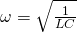.
• The charge and current in the circuit are given by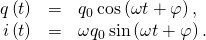### Conceptual Questions

Do Kirchhoff’s rules apply to circuits that contain inductors and capacitors?

yes

Can a circuit element have both capacitance and inductance?

In an LC circuit, what determines the frequency and the amplitude of the energy oscillations in either the inductor or capacitor?

The amplitude of energy oscillations depend on the initial energy of the system. The frequency in a LC circuit depends on the values of inductance and capacitance.

### Problems

A 5000-pF capacitor is charged to 100 V and then quickly connected to an 80-mH inductor. Determine (a) the maximum energy stored in the magnetic field of the inductor, (b) the peak value of the current, and (c) the frequency of oscillation of the circuit.

The self-inductance and capacitance of an LC circuit are 0.20 mH and 5.0 pF. What is the angular frequency at which the circuit oscillates?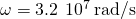What is the self-inductance of an LC circuit that oscillates at 60 Hz when the capacitance is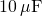?

In an oscillating LC circuit, the maximum charge on the capacitor is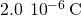and the maximum current through the inductor is 8.0 mA. (a) What is the period of the oscillations? (b) How much time elapses between an instant when the capacitor is uncharged and the next instant when it is fully charged?

a.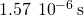; b.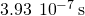The self-inductance and capacitance of an oscillating LC circuit are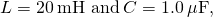respectively. (a) What is the frequency of the oscillations? (b) If the maximum potential difference between the plates of the capacitor is 50 V, what is the maximum current in the circuit?

In an oscillating LC circuit, the maximum charge on the capacitor is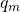. Determine the charge on the capacitor and the current through the inductor when energy is shared equally between the electric and magnetic fields. Express your answer in terms of, L, and C.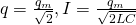In the circuit shown below,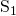is opened and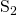is closed simultaneously. Determine (a) the frequency of the resulting oscillations, (b) the maximum charge on the capacitor, (c) the maximum current through the inductor, and (d) the electromagnetic energy of the oscillating circuit.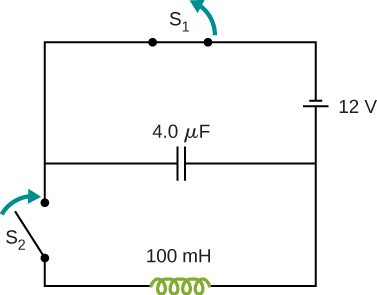An LC circuit in an AM tuner (in a car stereo) uses a coil with an inductance of 2.5 mH and a variable capacitor. If the natural frequency of the circuit is to be adjustable over the range 540 to 1600 kHz (the AM broadcast band), what range of capacitance is required?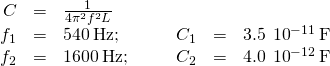### Glossary

LC circuit
circuit composed of an ac source, inductor, and capacitor